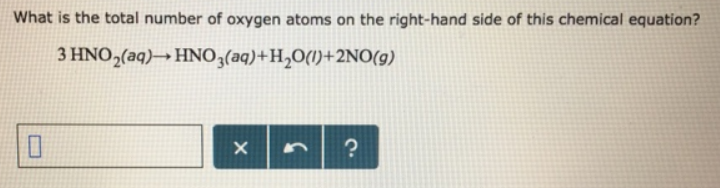# Problem: What is the total number of oxygen atoms on the right-hand side of this chemical equation? 3HNO2 (aq) → HNO3 (aq) +H2O (l) + 2NO (g)

🤓 Based on our data, we think this question is relevant for Professor Wu's class at OSU.

###### FREE Expert Solution###### Problem Details

What is the total number of oxygen atoms on the right-hand side of this chemical equation?

3HNO2 (aq) → HNO3 (aq) +H2O (l) + 2NO (g)